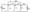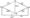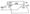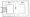# CSVTU Exam Papers – BE I Year – Basic Electrical Engineering – 2006-07

BE (I Year)

Examination -2006-07

Basic Electrical Engineering

Q1 (a) Use nodal analysis method to find currents In the various resistors of the circuit shown below.(b) Using the venin’s theorem and y/ V transformation ,find the current through the galvanometer of figure shown below:(c) Using Notton’s theorem ,find the current which would flow in a 15 Ohm resistor between point A and B in following figure(d) The case steel magnetic structure made of a bar section 2 cm x 2 cm is shown in figure .Determine the current that the 500 tun magnetizing coil on the left limb should carry so that a flush of2m Wb is produced in the right limb.Take and neglect leakageQ2(a) In a series parallel circuit , the parallel branch A and are in series with branch C. The impedances are

ZA=4 + fiZ„(4-f«3)

Zc=(2 + /)-4-H

If the current /c = (25 + j°) determine.

(j) Branch currents and voltage

(ii) Total voltages and –

(iv) Draw the pharos diagram.

(b) A 20 ohm resistor is connected in series with an index (R,L) i capacitor

(c) And an ammeter across a variable frequency supply . When the frequency 400HZ the current is at its maximum value c and the potential difference across the capacitor is 150V Calculate :

(i) The capacitance C of the capacitor

(ii)The resistance and inductane (R&L) of the inducator

Q3(a) Draw the phasor diagram of transformer (with resistance and leakage reactance) for inductive and capacitive loads

(b)  What do you mean by A1 day Efficiency ? A 5 KVA transformer has 35 W core loss and 40 W copper loss at futt load it operates at rated KVA and 0.8 power factor lagging for 6 hours, one half rated KVA and 0.5 power factor lagging for 12 hours and no load for 6 hours , what is all day efficiency ?

(c)  Derive the Emf  eqiiation of a transformer . Why the rating of transformer it expressed in kVA , not in kW ?

(d)Explain the open circuit test and short circuit test of a transformer

Q4 (a)Enlist various parts of a D.C. machine and explain the function of each part

(b) Describe the principle of operation of three phase induction motor (sq.cage type)

(c) Suggest the appropriate motor for following applications :

(i) Ceiling fan

(ii) Electric traction

(iii)Lathes

(iv) Cranes & hoists

(v) Blowers and fans

(vi)   Floor mill

(vii) Tape recorder

(ix) Tube well pump

Q5 (a) Describe the following torques and explain why they are required in elecricla measure in system:

(i) Deflecting torque

(ii) Controlling torque

(iii) Damping torque

(b)  Explain the working principle of single phase wattmeter.

(c)  Draw a’neat diagram of PMMC instrument and explain .its working